Courses

# NCERT Exemplar: States of Matter: Gases & Liquids Notes | EduRev

## JEE : NCERT Exemplar: States of Matter: Gases & Liquids Notes | EduRev

The document NCERT Exemplar: States of Matter: Gases & Liquids Notes | EduRev is a part of the JEE Course Chemistry for JEE.
All you need of JEE at this link: JEE

MULTIPLE CHOICE QUESTIONS

Q.1. A person living in Shimla observed that cooking food without using pressure cooker takes more time. The reason for this observation is that at high altitude:
(i) pressure increases
(ii) temperature decreases
(iii) pressure decreases
(iv) temperature increases

Ans. (iii)
Solution.
At high altitudes, pressure is low. Hence, boiling takes place at lower temperature and therefore, cooking takes more time. In pressure cooker, pressure is high and hence boiling point increases.

Q.2. Which of the following property of water can be used to explain the spherical shape of rain droplets?
(i) viscosity
(ii) surface tension
(iii) critical phenomena
(iv) pressure
Ans. (ii)
Solution.
Due to surface tension, the surface of the water drops is under tension and tends to take
spherical shape to reduce the tension.

Q.3. A plot of volume (V ) versus temperature (T) for a gas at constant pressure is a straight line passing through the origin. The plots at different values of pressure are shown in Figure. Which of the following order of pressure is correct for this gas?
(i) p1 > p2 > p3 > p4
(ii) p1 = p2 = p3 = p4
(iii) p1 < p2 < p3 < p4
(iv) p1 < p2 = p3 < p4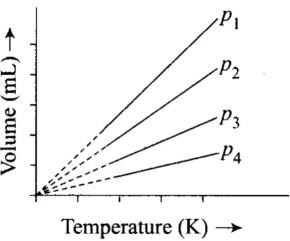Ans. (iii)
Solution.
At a particular temperature pV= constant.
Thus,
P1V= P2V= P3V= P4V4
As V1> V2 > V3 > V4, therefore, P1 <P2 < P3 < P4.

Q.4. The interaction energy of London force is inversely proportional to sixth power of the distance between two interacting particles but their magnitude depends upon
(i) charge of interacting particles
(ii) mass of interacting particles
(iii) polarisability of interacting particles
(iv) strength of permanent dipoles in the particles.

Ans. (iii)
Solution.
London dispersion forces operate only over very short distance. The energy of interaction varies as 1/(distance between two interacting particles)6 Large or more complex are the molecules, greater is the magnitude of London forces. This is obviously due to the fact that the large electron clouds are easily distorted or polarised.
Hence, greater the polarisability of the interacting particles, greater is the magnitude of the interaction energy.

Q.5. Dipole-dipole forces act between the molecules possessing permanent dipole.
Ends of dipoles possess ‘partial charges’. The partial charge is
(i) more than unit electronic charge
(ii) equal to unit electronic charge
(iii) less than unit electronic charge
(iv) double the unit electronic charge

Ans. (iii)
Solution.
Partial charge is a small charge developed by displacement of electrons. It is less than unit electronic charge and is represented as δ+ or δ

Q.6. The pressure of a 1:4 mixture of dihydrogen and dioxygen enclosed in a vessel is one atmosphere. What would be the partial pressure of dioxygen?
(i) 0.8×105 atm
(ii) 0.008 Nm–2
(iii) 8×104 Nm–2
(iv) 0.25 atm
Ans. (iii)
Solution.
Let the number of moles of dihydrogen and dioxygen be 1 and 4.
Mole fraction of O= 4/5.
Partial pressure of dioxygen = Mole fraction x Total pressure
= 4/5 x 1 = 0.8 atm = 0.8 x 105 Nm-2
= 8 x 10Nm-2

Q.7. As the temperature increases, average kinetic energy of molecules increases. What would be the effect of increase of temperature on pressure provided the volume is constant?
(i) increases
(ii) decreases
(iii) remains same
(iv) becomes half

Ans. (i)
Solution.
At constant volume, as the temperature is increased, pressure also increases.

Q.8. Gases possess characteristic critical temperature which depends upon the magnitude of intermolecular forces between the particles. Following are the critical temperatures of some gases.

 Gases H2 He O2 N2 Critical temperature in Kelvin 33.2 5.3 154.3 126

From the above data what would be the order of liquefaction of these gases?
Start writing the order from the gas liquefying first
(i) H2, He, O2, N2
(ii) He, O2, H2, N2
(iii) N2, O2, He, H2
(iv) O2, N2, H2, He
Ans. (iv)
Solution.
Higher the critical temperature, more easily is the gas liquefied. Hence, order of liquefaction starting with the gas liquefying first will be: 02, N2, H2, He.

Q.9. What is SI unit of viscosity coefficient (η)?
(i) Pascal
(ii) Nsm–2
(iii) km–2 s
(iv) N m–2

Ans. (ii)
Solution.
The SI unit of viscosity coefficient (η) is Nm-2 s or Nsm-2.
As we know that,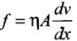Where,
= force
η = viscosity coefficient
Substitute SI units of f = N, dx = m, A = m2 and v = ms-1 in above equation, we get,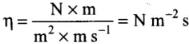Hence, the SI unit of η = is Nsm-2.

Q.10. Atmospheric pressures recorded in different cities are as follows:

 Cities Shimla Bangalore Delhi Mumbai p in N/m2 1.01 x 105 1.2 x 105 1.02 x 105 1.21 x 105

Consider the above data and mark the place at which liquid will boil first.
(i) Shimla
(ii) Bangalore
(iii) Delhi
(iv) Mumbai
Ans.
(i)
Solution.
Shimla has lowest atmospheric pressure, hence liquid will boil first in this city. Boiling of a liquid takes place when the vapour pressure becomes equal to the atmospheric pressure.

Q.11. Which curve in Figure represents the curve of ideal gas?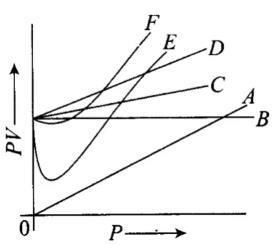(i) B only
(ii) C and D only
(iii) E and F only
(iv) A and B only

Ans. (a)
Solution.
For curve B, value of PV is constant and for an ideal gas plot of PV vs P is a straight line.

Q.12. Increase in kinetic energy can overcome intermolecular forces of attraction. How will the viscosity of liquid be affected by the increase in temperature?
(i) Increase
(ii) No effect
(iii) Decrease
(iv) No regular pattern will be followed

Ans. (iii)
Solution.
Intermolecular force of liquid decreases with increase in temperature, hence viscosity of liquid also decreases. However, some exceptions are there like liquid proteins and liquid sulphur.

Q.13. How does the surface tension of a liquid vary with increase in temperature?
(i) Remains same
(ii) Decreases
(iii) Increases
(iv) No regular pattern is followed

Ans. (ii)
Solution.
Surface tension of a liquid decreases with increase in temperature due to less forces of attraction between the molecules.

MORE THAN ONE CORRECT ANSWER TYPE

In the following questions two or more options may be correct.
Q.14. With regard to the gaseous state of matter which of the following statements are correct?
(i) Complete order of molecules
(ii) Complete disorder of molecules
(iii) Random motion of molecules
(iv) Fixed position of molecules
Ans. (ii, iii)
Solution.
In gaseous state, molecules are in a state of random motion, i.e., it is the state in which molecules are disorderly arranged. Gaseous state has higher entropy than the liquid as well as solid.

Q.15. Which of the following figures does not represent 1 mole of dioxygen gas at STP?
(i) 16 grams of gas
(ii) 22.7 litres of gas
(iii) 6.022 × 1023 dioxygen molecules
(iv) 11.2 litres of gas

Ans. (i,iv)
Solution.
1 mole of dioxygen represents 32 g of O2, 22.7 L of O2 or 6.022 x 1023 molecules of O2 gas.

Q.16. Under which of the following two conditions applied together, a gas deviates most from the ideal behaviour?
(i) Low pressure
(ii) High pressure
(iii) Low temperature
(iv) High temperature
Ans. (ii, iii)
Solution.
A gas which obeys the ideal gas equation, p V = nRTunder all conditions of temperature and pressure is called an ‘ideal gas’.
However, there is no gas which obeys the ideal gas equation under all conditions of temperature and pressure. Hence, the concept of ideal gas is only theoretical or hypothetical. The gases are found to obey the gas laws fairly well when the pressure is low or the temperature is high.
Such gases are, therefore, known as ‘real gases’. All gases are real gases. Hence, at high pressure and low temperature, a real gas deviates most from ideal behaviour.

Q.17. Which of the following changes decrease the vapour pressure of water kept in a sealed vessel?
(i) Decreasing the quantity of water
(iii) Decreasing the volume of the vessel to one-half
(iv) Decreasing the temperature of water
Ans. (ii, iv)
Solution.
Vapour pressure does not depend upon the quantity of water or size of the vessel. It decreases on adding salt to water or decreasing the temperature of water.

Q.18. If 1 gram of each of the following gases are taken at STP, which of the gases will occupy
(a) greatest volume and
(b) smallest volume?

CO, H2O, CH4, NO
Ans. Molar volume of a gas is volume occupied by 1 mole of gas at STP (273.15 K. and 1 bar pressure) and is equal to 22700 mL
28 g of CO occupy volume = 22700 mL
∴1 g of CO occupies volume =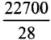mL at STP
Similarly,
1 g of H20 occupies volume =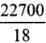mL at STP
1 g of CH4 occupies volume =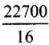mL at STP
1 g of NO occupies volume =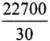mL at STP
(a) CH4 occupies greatest volume.
(b) NO occupies smallest volume.

Q.19. Physical properties of ice, water and steam are very different. What is the chemical composition of water in all the three states.
Ans. The chemical composition of water remains the same in all the physical states, i.e., solid, liquid and gas.

Q.20. The behaviour of matter in different states is governed by various physical laws. According to you what are the factors that determine the state of matter?
Ans. Pressure, temperature, mass and volume are the factors that determine the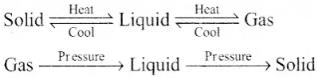Q.21. Use the information and data given below to answer the questions (a) to (c):

• Stronger intermolecular forces result in higher boiling point.
• Strength of London forces increases with the number of electrons in the molecule.
• Boiling point of HF, HCl, HBr and HI are 293 K, 189 K, 206 K and 238 K respectively.
(a) Which type of intermolecular forces are present in the molecules HF, HCl, HBr and HI?
(b) Looking at the trend of boiling points of HCl, HBr and HI, explain out of dipole-dipole interaction and London interaction, which one is predominant here.
(c) Why is boiling point of hydrogen fluoride highest while that of hydrogen chloride lowest?

Ans. (a) All the given molecules viz. HF, HCl, HBr and HI have permanent di-poles. Hence, all of them possess dipole-dipole and London forces. HF in addition to dipole-dipole and London forces also has hydrogen bonding.
(b) Electronegativity of Cl, Br and I is in the order: Cl > Br > I. Therefore, polar character and hence dipole-dipole interactions should be in the order HCl > HBr > HI. But boiling points are found to be in the order HCl < HBr < HI. This shows that London forces are predominant. This is because London forces increase as the number of electrons in the molecule increases. In this case, the number of electrons increases from HCl to HI.
(c) Due to very high electronegativity of F, HF is most polar and also there is hydrogen bonding present in it. Hence, it has the highest boiling point.

Q.22. What will be the molar volume of nitrogen and argon at 273.15K and 1 atm?
Ans. When temperature and pressure of a gas are 273.15 K (or 0°C) and 1 atm(or 1 bar or 105 pascal), such conditions are called standard temperature and pressure conditions (STP). Under these conditions, the volume occupied by 1 mole of each and every gas is 22.4 L. Hence, the molar volume of N2 and Ar at 273.15 K and 1 atm is 22,4 L.

Q.23. A gas that follows Boyle’s law, Charle’s law and Avogadro’s law is called an ideal gas. Under what conditions a real gas would behave ideally?
Ans. At high temperature and low pressure, the gases behave ideally since the two postulates of kinetic theory of gases are true under these conditions.
(i) The volume of a molecule of a gas is negligible as compared to its complete volume.
(ii) There is negligible force of attraction between the molecules of a gas.

Q.24. Two different gases ‘A’ and ‘B’ are filled in separate containers of equal capacity under the same conditions of temperature and pressure. On increasing the pressure slightly the gas ‘A’ liquefies but gas B does not liquify even on applying high pressure until it is cooled. Explain this phenomenon.
Ans. Gas ‘A’ is at critical temperature and therefore liquefies. Gas ‘B’ is at a temperature higher than critical temperature and therefore, does not liquefy even on applying high pressure.

Q.25. Value of universal gas constant (R) is same for all gases. What is its physical significance?
Ans. From the gas equation,
pV = nRT, R = pV/nT
If p is measured in Pascal, V in m3 n in mol and T in Kelvin, then units of R are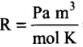= Pa m3 K-1 mol-1 or J mol-1 K-1
Since Joule is the unit of work, R represents work done per mole per Kelvin.

Q.26. One of the assumptions of kinetic theory of gases states that “there is no force of attraction between the molecules of a gas.” How far is this statement correct?Is it possible to liquefy an ideal gas? Explain.
Ans. The statement that there is no force of attraction between the molecules of a gas is true at low pressure and high temperature only. If the statement is true under all conditions of temperature and pressure, it will not be possible to liquefy an ideal gas.

Q.27. The magnitude of surface tension of liquid depends on the attractive forces between the molecules. Arrange the following in increasing order of surface tension : water, alcohol (C2H5OH) and hexane [CH3(CH2)4CH3)].

Ans. In the above given molecules, only hexane [CH3(CH2)4CH3] is a nonpolar molecule in which only London dispersion forces exist. These forces are very weak while both water and ethanol are polar molecules in which dipole- dipole interactions as well as H-bonding exists.
However, since H-bonding interactions are much stronger in water than ethanol, therefore, it possesses stronger intermolecular forces than alcohol and hexane. Hence, the increasing order of surface tension is
Hexane < Alcohol < Water

Q.28. Pressure exerted by saturated water vapour is called aqueous tension. What correction term will you apply to the total pressure to obtain pressure of dry gas?
Ans. Whenever a gas is collected over water, it is moist and saturated with water vapour which exerts their own pressure. The pressure due to water vapour is called aqueous tension. Thus, the total pressure of the gas (pmoist gas) is
Pmoist gas = Pdrygas
Thus Pdrygas  is given as
Pdrygas = Pmoist gas – aqueous tension. Hence, the correction term applied to the total pressure of the gas in order to obtain pressure of dry gas is Pmoist gas – aqueous tension.

Q.29. Name the energy which arises due to motion of atoms or molecules in a body. How is this energy affected when the temperature is increased?
Ans. The energy generated due to motion of atoms or molecules in a body is thermal energy. It is measured as average kinetic energy of molecules. It increases with increase in temperature.
K. E ∝ T

Q.30. Name two intermolecular forces that exist between HF molecules in liquid state.
Ans. (i) Dipole-dipole interactions
(ii) Hydrogen bonding

Q.31. One of the assumptions of kinetic theory of gases is that there is no force of attraction between the molecules of a gas. State and explain the evidence that shows that the assumption is not applicable for real gases.
Ans. Real gases can be liquefied on cooling and compressing which shows that there are forces of attractions between gaseous molecules.

Q.32. Compressibility factor, Z,  of a gas is given as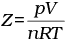(i) What is the value of Z for an ideal gas?
(ii) For real gas what will be the effect on value of Z above Boyle’s temperature?
Ans. (i) For ideal gas, Z = 1.
(ii) For a real gas, above Boyle’s temperature, gas shows positive deviation and hence Z > 1

Q.33. The critical temperature (Tc) and critical pressure ( pc ) of CO2 are 30.98°C and 73 atm respectively. Can CO2 (g) be liquefied at 32°C and 80 atm pressure?
Ans. C02 gas cannot be liquefied at 32°C by applying a pressure of 80 atm. This is because the temperature is higher than critical temperature of C02.

Q.34. For real gases the relation between p, V and T is given by van der Waals equation: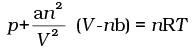where ‘a’ and ‘b’ are van der Waals constants, ‘nb’ is approximately equal to the total volume of the molecules of a gas. ‘a’ is the measure of magnitude of intermolecular attraction.
(i) Arrange the following gases in the increasing order of ‘b’. Give reason.

O2,  CO2,  H2,  He
(ii) Arrange the following gases in the decreasing order of magnitude of ‘a’.

Give reason.
CH4,  O2,  H2
Ans. (i) As ‘b’ represents molar volume occupied by the gas molecules, greater the size of the molecules, greater is the volume occupied by 1 mole of molecules. The size and hence the value of ‘b’ increase in the order: H2 < He < O2 < C02.
As all the given molecules are nonpolar, the magnitude of intermolecular attractions and hence the value of ‘a’ increases with the increase in number of electrons in the molecule, i.e., in the order: CH4 > 02 > H2. (Greater the number of electrons, greater is the size of electron cloud, greater is the polarization of the molecule, greater is the attraction).

Q.35. The relation between pressure exerted by an ideal gas (pideal) and observed pressure (preal) is given by the equation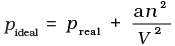If pressure is taken in Nm–2, number of moles in mol and volume in m3, Calculate the unit of ‘a’.
What will be the unit of ‘a’ when pressure is in atmosphere and volume in dm3?
Ans.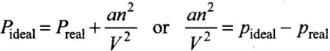If pressure is in Nm-2, volume in m3, n is in mol, then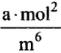= Nm-2 or a = Nmmol-2
If pressure is in atm and volume in dm3, then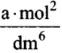= atm or a = atm dmmol -2

Q.36. Name two phenomena that can be explained on the basis of surface tension.
Ans. (i) Spherical shape of liquid drops.
(ii) Cleansing action of soaps and detergents.
(iii) Capillary action → Rise and dip of liquid in the column of capillary.

Q.37. Viscosity of a liquid arises due to strong intermolecular forces existing between the molecules. Stronger the intermolecular forces, greater is the viscosity. Name the intermolecular forces existing in the following liquids and arrange them in the increasing order of their viscosities. Also give reason for the assigned order in one line.
Water, hexane (CH3CH2CH2CH2CH2CH3), glycerine (CH2OH CH(OH) CH2OH)
Ans. The increasing order of viscosity is Hexane < Water < Glycerine
In hexane, there are only London forces, whereas in water and glycerine there is hydrogen bonding which is stronger in case of glycerine (because of the presence of three -OH groups) than that in water.

Q.38. Explain the effect of increasing the temperature of a liquid, on intermolecular forces operating between its particles, what will happen to the viscosity of a liquid if its temperature is increased?
Ans. If the temperature is increased, the intermolecular forces become weak and kinetic energy increases. As the temperature increases the kinetic energy can overcome intermolecular forces, hence viscosity decreases and liquids can flow easily.

Q.39. The variation of pressure with volume of the gas at different temperatures can be graphically represented as shown in Figure.
On the basis of this graph answer the following questions.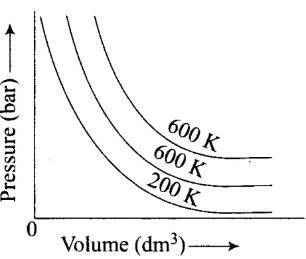(i) How will the volume of a gas change if its pressure is increased at constant temperature?
(ii) At a constant pressure, how will the volume of a gas change if the temperature is increased from 200K to 400K?
Ans. (i) The volume of a gas will decrease if the pressure on the gas is increased keeping the temperature constant.
(ii) The volume of a gas increases if the temperature is increased keeping the pressure constant.

Q.40. Pressure versus volume graph for a real gas and an ideal gas are shown in Figure. Answer the following questions on the basis of this graph.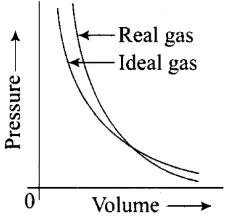(i) Interpret the  behaviour of real gas with respect to ideal gas at low pressure.
(ii) Interpret the behaviour of real gas with respect to ideal gas at high pressure.
(iii) Mark the pressure and volume by drawing a line at the point where real gas behaves as an ideal gas.

Ans. (i) At low pressure, the two* curves almost coincide. This shows that at low pressure, the real gases show very small deviation from ideal behaviour.
(ii) At high pressure, the curves are far apart. This shows that real gases show large deviations at high pressure.
(iii) At point A, where the two curves intersect each other, the real gas behaves exactly like ideal gas and the pressure and volume corresponding to this point are P1 and V1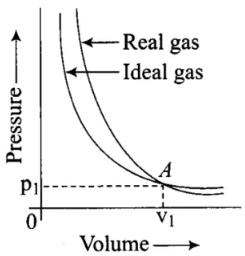MATCHING TYPE

Q.41. Match the graphs between the following variables with their names :

 Graphs Names (i) Pressure vs temperature graph at constant molar volume. (a) Isotherms (ii) Pressure vs volume graph at constant temperature. (b) Constant temperature curve (iii) Volume vs temperature graph at constant pressure. (c) Isochores (d) Isobars

Ans. (i) →(c); (ii) → (a); (iii) → (d)
(i) Pressure vs temperature graph at constant volume – Isochores
(ii) Pressure vs volume graph at constant temperature – Isotherms
(iii) Volume vs temperature graph at constant pressure – Isobars

Q.42. Match the following gas laws with the equation representing them.

 (i) Boyle’s law (a) V ∝ n at constant T and p (ii) Charle’s law (b) pTotal = p1 + p2 + p3+...... at constant  T, V (iii) Dalton’s law (c) pV/T = Constant (iv) Avogadro law (d) V ∝ T at constant n and p (e) p ∝ 1/V at constant n and T

Ans. (i) → (e); (ii) → (d); (iii) → (b); (iv) → (a).
(i) Boyle’s law: P ∝ 1/V at constant n and T.
(ii) Charles’ law: V∝ T at constant n and P.
(iii) Dalton’s law: P1 + P2 + P3 .............. Ptotal at constant T and V.
(iv) Avogadro’s law: V ∝ n at constant T and P.

Q.43. Match the following graphs of ideal gas with their coordinates:

 Graphical representation x and y co-ordinates (i)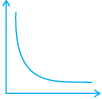(a) pV  vs. V (ii)(b) p vs. V (iii)(c)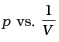Ans. (i) → (b), (ii) → (c), (iii) → (a)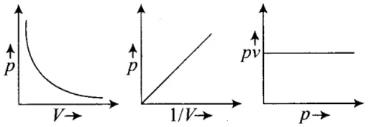ASSERTION AND REASON TYPE

In the following questions a statement of Assertion (A) followed by a statement of Reason (R) is given. Choose the correct option out of the choices given below each question.
Q.44. Assertion (A): Three states of matter are the result of balance between intermolecular forces and thermal energy of the molecules.
Reason (R): Intermolecular forces tend to keep the molecules together but thermal energy of molecules tends to keep them apart.
(i) Both A and R are true and R is the correct explanation of A.
(ii) Both A and R are true but R is not the correct explanation of A.
(iii) A is true but R is false.
(iv) A is false but R is true.

Ans. (i)
Solution.
States of matter of a substance depend on the intermolecular forces.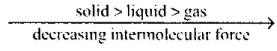Intermolecular forces keep the molecules together while thermal energy of molecules tends to keep them apart.

Q.45. Assertion (A): At constant temperature, pV vs V plot for real gases is not a straight line.
Reason (R) : At high pressure all gases have Z > 1 but at intermediate pressure most gases have Z < 1.
(i) Both A and R are true and R is the correct explanation of A.
(ii) Both A and R are true but R is not the correct explanation of A.
(iii) A is true but R is false.
(iv) A is false but R is true.

Ans. (ii)
Solution.
PV versus Fplot is straight line for ideal gases but not for real gases.
At high pressure, there is positive deviation from ideal behaviour (Z < 1) but at intermediate or moderate pressure, the real gases pass negative deviation from ideal behavior (Z < 1).

Q.46. Assertion (A): The temperature at which vapour pressure of a liquid is equal to the external pressure is called boiling temperature.
Reason (R) : At high altitude atmospheric pressure is high.
(i) Both A and R are true and R is the correct explanation of A.
(ii) Both A and R are true but R is not the correct explanation of A.
(iii) A is true but R is false.
(iv) A is false but R is true.

Ans. (iii)
The temperature at which the vapour pressure of a liquid becomes equal to the external pressure is called its boiling point. Atmospheric pressure decreases with increase in altitude.

Q.47. Assertion (A): Gases do not liquefy above their critical temperature, even on applying high pressure.
Reason (R) : Above critical temperature, the molecular speed is high and intermolecular attractions cannot hold the molecules together because they escape because of high speed.
(i) Both A and R are true and R is the correct explanation of A.
(ii) Both A and R are true but R is not the correct explanation of A.
(iii) A is true but R is false.
(iv) A is false but R is true.

Ans. (i)
Solution.
According to Andrews, a real gas cannot be liquefied‘above critical ‘ temperature, whatever pressure is applied. Intermolecular force of gas molecules is low above critical temperature.

Q.48. Assertion (A): At critical temperature liquid passes into gaseous state imperceptibly and continuously.
Reason (R) : The density of liquid and gaseous phase is equal to critical temperature.
(i) Both A and R are true and R is the correct explanation of A.
(ii) Both A and R are true but R is not the correct explanation of A.
(iii) A is true but R is false.
(iv) A is false but R is true.

Ans. (i)
Solution.
At critical state, surface tension of liquid is zero hence liquid passes into gaseous state imperceptibility and continuously . At this stage , the liquid and vapour phase becomes equal.

Q.49. Assertion (A): Liquids tend to have maximum number of molecules at their surface.
Reason (R) : Small liquid drops have spherical shape.
(i) Both A and R are true and R is the correct explanation of A.
(ii) Both A and R are true but R is not the correct explanation of A.
(iii) A is true but R is false.
(iv) A is false but R is true.

Ans. (iv)
Solution.
Liquid tends to acquire minimum surface area due to surface tension. Thus, small liquid drops are spherical. In spherical shape, surface area is minimum.

Q.50. Isotherms of carbon dioxide at various temperatures are represented in Figure.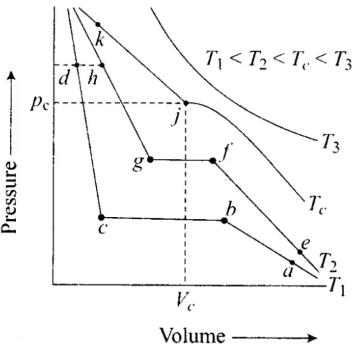Answer the following questions based on this figure.
(i) In which state will CO2 exist between the points a and b at temperature
T1?
(ii) At what point will COstart liquefying when temperature is T1?
(iii) At what point will CO2 be completely liquefied when temperature is T2.
(iv) Will condensation take place when the temperature is T3.
(v) What portion of the isotherm at T1 represent liquid and gaseous CO2 at equilibrium?

Ans. (i) At T1 (between points ‘a’ and ‘b’) C02 exists in gaseous state.
(ii) At point b, C02 starts liquefying at T1
(iii) At temperature T2, at point ‘g’ C02 will be completely liquefied.
(iv) No condensation at T3 since T3 > Tc.
(iv) At Tliquid and gaseous C02 are at equilibrium between b and c.

Q.51. The variation of vapour pressure of different liquids with temperature is shown in Figure
(i) Calculate graphically boiling points of liquids A and B.
(ii) If we take liquid C in a closed vessel and heat it continuously. At what temperature will it boil?
(iii) At high altitude, atmospheric pressure is low (say 60 mm Hg). At what temperature liquid D boils?
(iv) Pressure cooker is used for cooking food at hill station. Explain in terms of vapour pressure why is it so?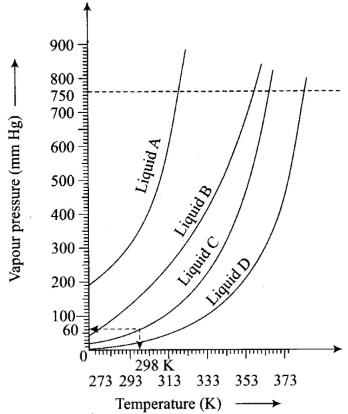Ans. (i) Boiling point of liquid A ≃315 K, B ≃ 345 K
(ii) In a closed vessel, liquid C will not boil because pressure inside keeps on increasing.
(iii) Temperature corresponding to 60 mm ≃ 313 K.
(iv) A liquid boils when its vapour pressure becomes equal to atmospheric pressure. At hill station, atmospheric pressure is low. Therefore, liquid boils at a lower temperature and cooking is not perfect. In a pressure cooker the pressure inside increases and the liquid boils at a higher temperature.

Q.52. Why does the boundary between liquid phase and gaseous phase disappear on heating a liquid upto critical temperature in a closed vessel? In this situation what will be the state of the substance?
Ans. When a liquid is heated up to its critical temperature in a closed vessel, it does not pass through a two phase region and substances remain in one phase. There is a continuity between a gaseous and liquid state. The term fluid is used for either a liquid or a gas to recognize this continuity. Liquid and gas can be distinguished only when the fluid is below its critical temperature and the surfaces separating them can be seen. At critical temperature, liquid passes into gaseous state continuously and the surface separating the two phases disappears. A gas below the critical temperature can be liquefied by applying pressure.

Q.53. Why does sharp glass edge become smooth on heating it upto its melting point in a flame? Explain which property of liquids is responsible for this phenomenon.

Ans. On heating the glass, it melts and takes up rounded shape at the edges which has minimum surface area. This is due to the property of surface tension of liquids.

Q.54. Explain the term ‘laminar flow’. Is the velocity of molecules same in all the layers in laminar flow? Explain your answer.

Ans. When a liquid flows over a fixed surface, the layer of molecules in the immediate contact of surface is stationary. The velocity of the upper layers increases as the distance of layers from the fixed layer increases.
This type of flow in which there is a regular gradation of velocity on passing from one layer to the next is called laminar flow.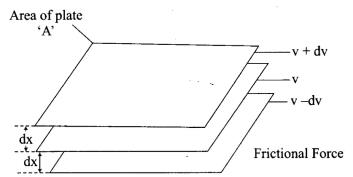In laminar flow, the velocity of molecules is not same in all the layers because every layer offers some resistance or friction to the layer immediately below it.

Q.55. Isotherms of carbon dioxide gas are shown in Figure. Mark a path for changing gas into liquid such that only one phase (i.e., either a gas or a liquid) exists at any time during the change. Explain how the temperature, volume and pressure should be changed to carry out the change.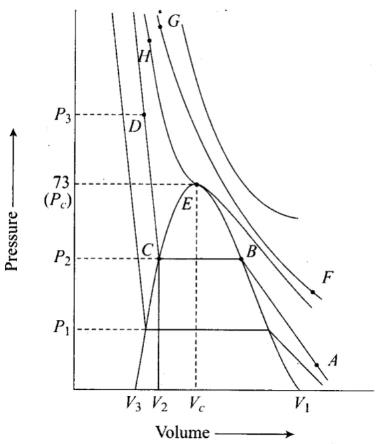Ans. In isotherm of carbon dioxide, it is possible to change a gas into liquid or a liquid into gas by a process in which always a single phase is present.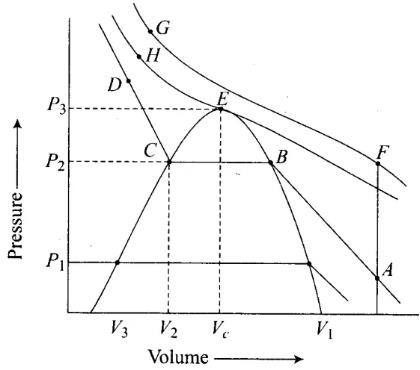If we move vertically from point A to F by increasing the temperature, then we can reach the point G by compressing the gas at constant temperature along this (isotherm at 31.1°C). Now we can move vertically downwards to D by lowering the temperature. As soon as we cross point H on critical isotherm, we get liquid. If process is carried out at critical temperature, substance always remains in one phase. Hence the path for the change is A → F → G → H → D.

Offer running on EduRev: Apply code STAYHOME200 to get INR 200 off on our premium plan EduRev Infinity!

## Chemistry for JEE

223 videos|452 docs|334 tests

,

,

,

,

,

,

,

,

,

,

,

,

,

,

,

,

,

,

,

,

,

;